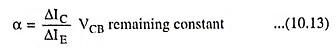## Current Amplification Factor:

There are two current amplification factors, the alpha factor (α) and the beta factor (β) defined below :

The alpha factor (α) is also called the current amplification factor and is the ratio of change in collector current (IC) to change in emitter current (IE).

In general,The beta factor (β) is the current gain factor (also called the transport factor) of a common emitter circuit and is defined as the ratio of change in collector current (IC) to change in base current (IB).

In general,

Scroll to Top